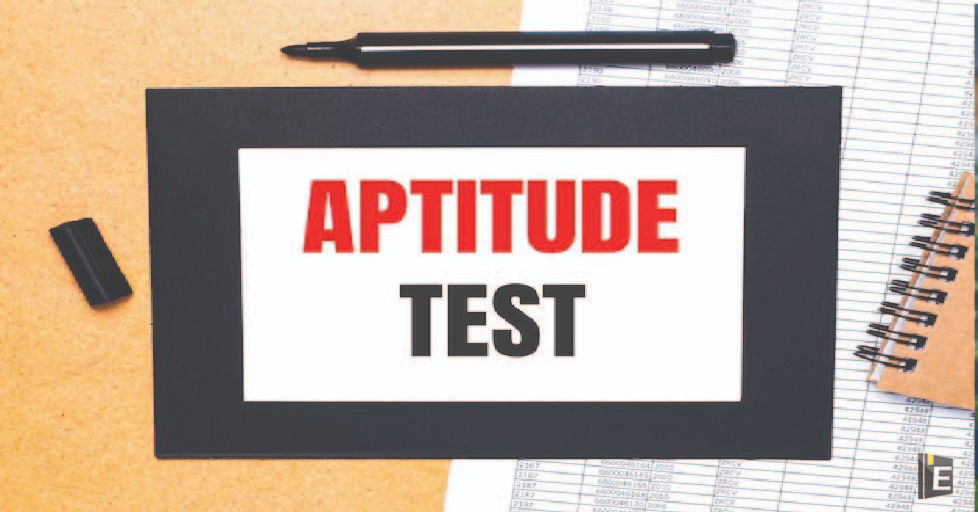• Save

# Aptitude Questions Quiz with Answers

NEWWelcome to Aptitude Questions Quiz with Answers," your one-stop destination for a wide range of aptitude questions and expert answers! Whether you're gearing up for a competitive exam, or job interview, or simply looking to enhance your problem-solving skills, this Aptitude Questions Quiz with Answers blog is designed to be your trusted companion on your journey towards success. Our team of experienced educators and subject-matter experts meticulously break down each question, providing step-by-step solutions that make complex problems easy to understand. You'll find tips, tricks, and strategies that empower you to tackle similar challenges with confidence.

## Aptitude Questions

Our Aptitude Questions Quiz with Answers blog is carefully curated to provide you with a diverse collection of aptitude questions that span various subjects, including mathematics, logical reasoning, verbal ability, and more. Each question is handpicked to challenge your intellect and help you sharpen your analytical thinking, making it an invaluable resource for students, job seekers, and lifelong learners alike.

Also, Read Latest Current Affairs Questions 2023: Current Affairs Today

"Put your knowledge to the test and excel with our General Knowledge Mock Test and Current Affairs Mock Test!"

## Aptitude Questions Quiz with Answers

Q :

Two vessels A and B contain spirit and water mixed in the ratio 5:2 and 7:6 respectively. Find the ratio in which these mixture be mixed to obtain a new mixture in vessel C containing spirit and water in the ratio 8:5?

(A) 9 : 7

(B) 2 : 3

(C) 7 : 5

(D) 5 : 7

(E) 7 : 9

Q :

Ten different letters of alphabet are given, words with 5 letters are formed from these given letters. Then, how many such words can be formed which have at least one letter repeated ?

(A) 69760

(B) 69970

(C) 67960

(D) 65760

(E) 69790

Q :

Water flows into a tank which is 200 m long and 150 m wide, through a pipe of cross-section (0.3m x 0.2m) at 20 km/h. In what time will the water level be 8 m?

(A) 450

(B) 500

(C) 200

(D) 250

(E) 350

Q :

A work is done by three person A, B and C. A alone takes 10 hours to complete the work but B and C working together takes 4 hours, for the completion of the same work. If all of them worked together and completed 14 times of the work, then how many hours have they worked?

(A) 42 hr

(B) 28 hr

(C) 40 hr

(D) 20 hr

(E) 45 hr

Q :

40% of the employees in a factory are workers. All the remaining employees are executives. The annual income of each worker is Rs. 39000. The annual income of each executive is Rs. 42000. What is the average annual income of all the employees in the factory together?

(A) 40800

(B) 41500

(C) 40000

(D) 38500

(E) 27000

Q :

In the given question, two equations numbered l and II are given. Solve both the equations and mark the appropriate answer.

I. x2– 37x + 330 = 0

II. y2– 28y + 195 = 0

(A) x > y

(B) x < y

(C) x ≥ y

(D) x ≤ y

(E) x = y or relationship between x and y cannot be established.

Q :

The average of 8 numbers is 38.4. Out of these numbers, the average of 7 number is 39.2. The 8th numbers

(A) 33.7

(B) 31.5

(C) 35.2

(D) 32.8

(E) 34.5

Q :

A bag contains 3 white balls and 2 black balls. Another bag contains 2 white and 4 black balls. A bag and a ball are picked random. The probability that the ball will be white is:

(A) 7/15

(B) 9/16

(C) 4/15

(D) 3/5

(E) 8/5

Q :

In the given question, two equations numbered l and II are given. Solve both the equations and mark the appropriate answer.

I. x2- 12x + 35 = 0

II. y2- 25y + 126 = 0

(A) x > y

(B) x < y

(C) x ≥ y

(D) x ≤ y

(E) x = y or relationship between x and y cannot be established.

Q :

In the given question, two equations numbered l and II are given. Solve both the equations and mark the appropriate answer.

I. x2– 35x + 294 = 0

II. y2– 68y + 1140 = 0

(A) x > y

(B) x < y

(C) x ≥ y

(D) x ≤ y

(E) x = y or relationship between x and y cannot be established.